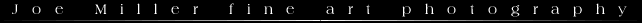## Depth-of-Field Calculator * **

To find the near, far and hyperfocal distance for a specific lens, enter the focal length (mm), aperture (f/number) and the object distance (whole or decimal number). The JavaScript program will calculate the near, far, depth and hyperfocal distances. The unit of measure for distances is feet. This can be overridden by selecting meters. Your MSIE and later Netscape browser will print the tables, others must print screen to the clipboard and print from some other application.
Depth-of-Field - Unit of Measure is meters.
Film
Format
Focal
Length
(mm)
f/No Object
Distance
Near
Distance
Far
Distance
Depth
of
Field
Hyperfocal
Distance
We Got
Buttons
Depth-of-Field Table for the above Focal Length
f/5.6 f/8 f/11 f/16 f/22 f/32 f/45
"Inf"
*The formulas used for this table.
**Javascript for the DOF table written by Michael C Gillett

MAXMIZING  THE USEFULNESS OF  LENS DEPTH OF FIELD  SCALES

Example and Definitions

Depth of field (DOF) refers to the area in front of and behind the point of focus that is also in acceptable focus.  Assume that you are using a 35mm camera with a 50mm lens at f/8 and have focused on a subject  15 feet away.  If you enter this information into the calculator above you would get the depth of field indicated  in the figure below: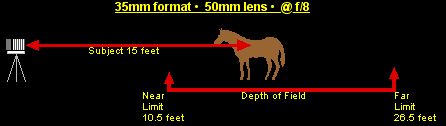The near distance (sometimes called the near limit) is the point closest to the camera that is in acceptable focus and the far distance (or far limit) is the point farthest from the camera in acceptable focus.  Of course, the  distance between these two points is the depth of field.   In the DOF table at the top of the page you are required to enter four variables.   All other things equal, each of these affects DOF as follows:

• F/stop or Aperture -- as you  stop down the lens  or decrease the size of  the aperture, you increase DOF.   For example,  f/8 provides greater DOF than  f/5.6,  f/11 more DOF than f/8 and so on.  For any given composition, this is the photographer's main DOF tool
• Lens Focal Length -- as focal length increases DOF decreases.  Wide angle lenses provide greater DOF than normal or telephoto lenses.
• Object Distance --  the greater the camera to subject distance the greater the DOF.  Close up photography involves dealing with shallow a DOF.
• Format -- the DOF formulas for the above table assume that focus is less critical for larger negatives because they are usually enlarged less.  (See the discussion of the  "circle of confusion" below.

• Unfortunately,  many modern autofocus and zoom lenses lack a DOF scale.  Older prime (nonzoom) manual focus and medium format lenses do have a scale such as the one shown below: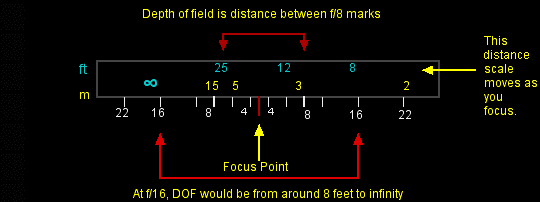Hyperfocal distance is the nearest point to the camera in acceptable focus if the lens is focused at infinity (i.e, the near limit).  If you focus at the hyperfocal distance, everything from one-half the hyperfocal distance to infinity will be in acceptable focus.  This is a technique often used by landscape photographers to maximize the depth of field.  This point can be easily found with lenses that have  DOF markings.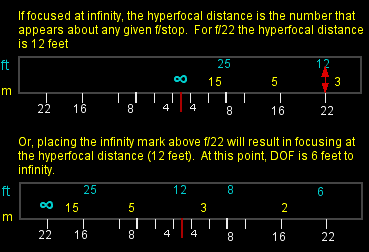What Does This Really Mean

The precision of the answers provided by the calculator, or any DOF table for that matter, can be a bit misleading.  In the above example, a far limit of  26.5  does not mean that an object at 26.4 feet would be in perfect focus while an object at 26.6 would be totally out of focus.  Focus gradually declines as you move in any direction from the point of focus at 15 feet.   Remember, the definition uses the term "acceptable".  I would like to think of 26.5 feet as a vicinity, not an absolute point.

Also, the word "acceptable" when referring to focus would seem to be rather subjective.  In other words, what I find  to be acceptable focus may not be acceptable to you.  Also, what I would find acceptable in a small enlargement might not be acceptable in a larger print.  However, the formulas used by the above calculator employ  an objective measure of focus called the "circle of confusion".  As a point of light becomes more and more out of focus, it increases in size to become a disk.  This disk is called the "circle of confusion" and its size (or diameter) can be used as  a measure of focus.  A point in the distance is considered to be in focus if its circle of confusion is equal to or below the specified size. Different size circles of confusion are used for each of the format sizes (35mm, 6x7, 4x5 and etc.) because the larger negatives require less enlargement.   The table above, in my opinion,  uses what are most likely generally accepted circles of confusion.  If you are handy with a spread sheet it is no great task to create DOF tables for your specific lenses using whatever DOF you desire.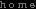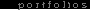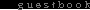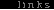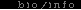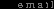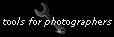All Text and Images © Joe Miller, 2004